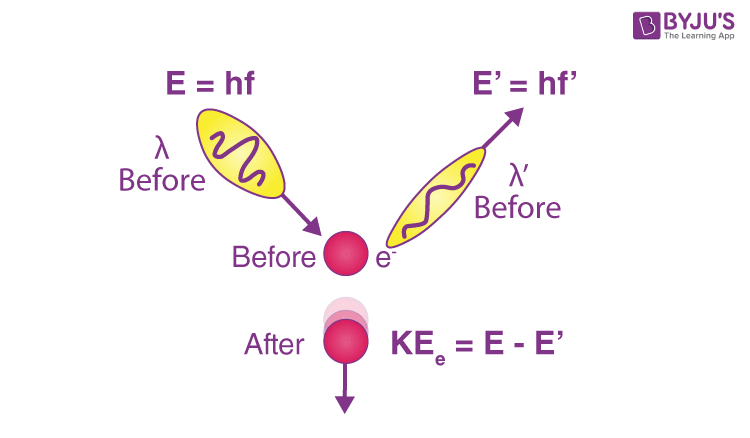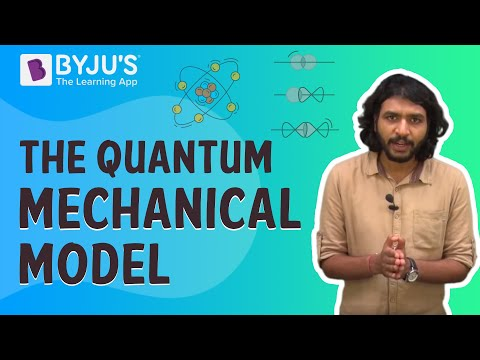Checkout JEE MAINS 2022 Question Paper Analysis : Checkout JEE MAINS 2022 Question Paper Analysis :

# Photon Momentum

## Understanding Photon

Photon is considered a type of elementary particle. It is the quantum of the electromagnetic field that includes electromagnetic radiation such as light and radio waves. The photon is categorized under the class of bosons. We understand that particles carry momentum and energy. However, photons have no mass, but there is evidence that electromagnetic radiation carries momentum. The behaviour of photons is explained using quantum mechanics.

Table of Content

## What is Momentum?

Momentum can be defined as the product of the mass and velocity of an object. This is derived from Newtonian mechanics. Momentum has magnitude and direction and therefore, it is a vector quantity.

The following formula is used for calculating the momentum of an object:

P = mxv

Where,

• m is the mass of the object
• v is the velocity of the object

The SI unit of momentum is kg.m/s and its dimensions are [MLT-1].

## What is Photon Momentum?

Photon momentum is also known as the momentum of a photon. The momentum of a photon is related to its wavelength, while the energy of a photon is proportionate to its frequency.

The below diagram is an illustration of the Compton Effect and the formula is:p = h/λ

Where,

• p is the momentum of the photon in kg.m/s
• h is the Planck’s constant (6.63×10-34 J.s)
• λ is the wavelength of a wave carrying the photon in m

When a photon is scattered by an electron, this effect is known as the Compton effect. During this effect, momentum, as well as energy, is conserved. When this occurs, the scattered photon will have less energy and momentum. This study helped Compton prove that the photons have momentum.

### Example for Photon Momentum

Example:

The wavelength of the light is given as 4×10-7 m. Determine the momentum of the photon of light.

Solution:

Given:

The wavelength of the light, λ = 4×10-7 m

We know that the Planck’s constant, h = 6.63×10-34 J.s

Substituting the values in the formula,

p = h/λ = 6.63×10-34/4×10-7 = 1.65×10-27 kg.m/s

Summary

• A quantum of light that has no mass is known as a photon.
• Momentum is the product of the mass and velocity of an object.
• Photon momentum is given: p = h/λ

The below video is an explanation of a quantum mechanical model:## Frequently Asked Questions – FAQs

### In the electromagnetic spectrum, which photon carries the most energy and momentum?

In the electromagnetic spectrum, gamma rays carry the most energy and momentum.

### Why is quantum mechanics important?

Quantum mechanics is important because it helps in understanding the electronic structure of a chemical compound at the theoretical level. Also, factors such as thermodynamics, kinetics, and other mechanisms are studied.

### What is the difference between Compton effect and photoelectric effect?

The difference between Compton effect and photoelectric effect is that the Compton effect is caused by the inelastic scattering of photons that are bound to the free electrons. Whereas, photoelectric effect is caused by the weakly bound electrons that are ejected from the surface of the material when the electromagnetic radiation interacts with the electrons.

### How much energy is in a photon?

In visible light, one photon contains 10-19 Joules.

### What is a photon equal to?

A photon is equal to 1 eV = 1.602×10-19 J.